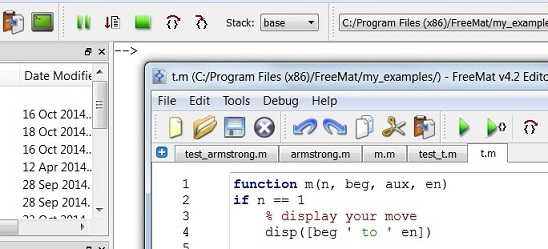# Programming Functions – Basic Concepts

We'll see how to program functions while in the development of sofware and it’s better if we understand their purpose and definition.

If you want to know how to create functions in Matlab, go to
custom functions in Matlab.

 A function in programming is the means by which someone who designs or uses a  program can execute a block of code which has two purposes: to finish a certain routine or task and/or to return processed values. A function is also considered a procedure in some programming languages.

On the other hand, software functions are commonly known as any term that is being used in referring to names of code blocks. Functions accept restrictions, they also return values, and they usually are maintained on a separate location from the code of the main program.Perhaps, the most common example of a programming function is a mathematical function.  Some reserved words, such as ‘log’ (for logartithm) and ‘tan’ (for tangent) are examples of mathematical functions.
Many times, there are special functions that work with ‘strings’ (alphanumerical characters) and time functions, just to name a few...

Simply expressed, functions in programs allow you to assign certain values where results can be calculated in a matter of seconds  (usually much less) while saving you from the task of doing the operations or computations manually.

On the declaration or calling of a function which has two or more parameters, the use of comma is needed to separate the different parameters.  Particularly in Matlab (or in any other of its similar open source counterparts, such as Scilab, GNU-Octave, FreeMat... etc.) one function declaration could look like this:

function [cm, kg] = anglo2metric(in, lb)
cm = in * 2.54;
kg = lb * 0.45359237;

You send parameters ‘in’ (for inches) and ‘lb’ (for pounds) to function ‘anglo2metric’. This function processes those numbers and return two values, in ‘cm’ (for centimeters) and ‘kg’ (for kilograms). You can choose the names of the input parameters and of the output values.

A coded function is used to return some value or information.  Functions (made by you or built-in) do calculations, sure, but they’re also useful to indicate some errors that they encounter, let’s say for debugging purposes.

On this page we’re not talking about any specific programming language but functions do exist in every computer language.

An example of script for PHP is the following:

<?php
function add_numbers(\$var1 = 0, \$var2 = 0, \$var3 = 0)
{
\$var4 = \$var1 + \$var2 + \$var3;
return \$var4;
}

echo “The result of 3 + 4 + 6 is {\$sum}
?>

After execution, you get:

The result of 3 + 4 + 6 is 13

Note that sometimes you need special characters (such as ‘{’ and ‘}’) to define your start/end of your function, or the reserved words ‘return’ or ‘endfunction’ to end that portion of code.

These sentences may look like a bunch of strange letters and numbers but these symbols actually account to make a certain task easier.  And that, for the moment, is what we want to stress.

From 'Programming Functions ' to home

From 'Programming Functions' to 'Basics of Programming'